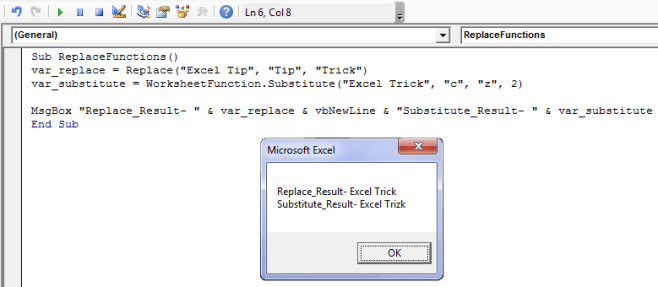# VBA Replace Function – How to Use in Excel

VBA Replace is a quite useful string function in Excel VBA. Functions like replace ease your tasks while dealing with strings.

As the name suggests the job of the Replace function is to substitute a set of characters in a string with a new set of characters.

In Excel VBA there are two functions that can be used for performing string replace operations. And today I will highlight and give examples of both these functions:## 1. VBA REPLACE Function

As I have foretold the Replace function simply replaces a set of characters from a string with another predefined set of characters.

The basic syntax of a VBA Replace function is as follows:

`=Replace( Source_string, Old_string, Replacement_string, [start, [count, [compare]]] )`

Here, ‘Source_string’ is the string from which we have to replace the characters.

‘Old_string’ is a set of characters or a string which is to be replaced.

‘Replacement_string’ is a set of characters with which the ‘Old_string’ is to be replaced.

‘start’ represents the numerical position in the ‘Source_string’ from which the search should begin. It is an optional parameter. If we omit this parameter then the search begins at position 1.

‘count’ is the number of occurrences of ‘Old_string’ to be replaced. It is an optional parameter. If its value is omitted then all the occurrences of ‘Old_string’ in the ‘Source_string’ will be replaced.

‘compare’ is also an optional parameter. It signifies the type of comparison algorithm to be used while Replace Function searches for the occurrences of ‘Old_string’ in the ‘Source_string’. In most cases, it is better to omit this value. But for advanced cases, the values of this parameter can be any one of these.

Parameter ValueDescription
vbBinaryCompare Use this in case of a Binary comparison.
vbTextCompare Use this in case of a Text to Text comparison
vbDatabaseCompare It uses the locale settings of a Database for comparison rather than straight text.

### Examples of VBA REPLACE Function

Example 1:

`Replace("Excel Tips", "Tips", "Trick")`

This statement would return “Excel Trick”.

Example 2:

`Replace("Excel VBA", "Tips", "Trick")`

This statement would return “Excel VBA” as here we have asked the Replace function to replace “Tips” but as you can see “Tips” text string is not present inside the Source string, hence the output will be Source string as it is.

Example 3:

`Replace("alligator", "a", "z", 2 )`

This statement will result in “alligztor” as here the Replace statement starts searching for the character ‘a’ after the second position in the source string and then replaces it with ‘z’ character.

Example 4:

`Replace("alligator", "a", "z", 1, 1)`

This statement would result in “zlligator” as here we have told the VBA Replace Statement to replace only one occurrence of ‘a’ with ‘z’.

Example 5:

`Replace("Alligator", "a", "z", 1, , vbTextCompare)`

The use of vbTextCompare in this statement tells the Replace Statement to ignore the difference between Upper Case and Lower Case characters hence this statement results in: “zalligztor”.

## 2. Excel VBA SUBSTITUTE Function:

The Excel VBA SUBSTITUTE function is very similar to the Replace Statement. The syntax of both these functions is almost the same, the results they deliver are the same too.

The point that is very important while using the Substitute function is that: this function is not readily available in the VBA functions but under the VBA WorksheetFunctions. See, the syntax of the Substitute function below:

`WorksheetFunction.Substitute("Source_string", "Old_string", "Replacement_string", Instance_num)`

Here also the ‘Source_string’ is the string from which we have to replace the characters.

‘Old_string’ is a set of characters which are to be replaced.

‘Replacement_string’ is a set of characters with which the ‘Old_string’ is to be replaced.

‘Instance_num’ is an integer parameter that specifies which occurrence of ‘Old_string’ you want to replace with ‘Replacement_string’. This is an optional argument. If it is omitted then all the occurrences of ‘Old_string’ will be replaced.

### Examples of VBA SUBSTITUTE Function:

Example 1:

`Substitute ("Excel Tips", "Tips", "Trick")`

This statement would return “Excel Trick”.

Example 2:

`Substitute ("H:\SomeFolder\AnotherFolder\SomeFile.txt", "\", "*", 3)`

This statement returns: “H:\SomeFolder\AnotherFolder*SomeFile.txt”. As here only the third instance of “\” character is replaced by “*”.

## Excel Macro Using Above Functions:

I have created an Excel Macro in which I have used both the above functions. The macro is quite simple and self-explanatory.The macro is as under:

`Sub ReplaceFunctions()var_replace = Replace("Excel Tip", "Tip", "Trick")var_substitute = WorksheetFunction.Substitute("Excel Trick", "c", "z", 2)MsgBox "Replace_Result- " & var_replace & vbNewLine & "Substitute_Result- " & var_substituteEnd Sub`

So, these are some of the VBA string Replace functions that can be used in Excel Macros.About Ankit Kaul

Ankit is the founder of Excel Trick. He is tech Geek who loves to sit in front of his square headed girlfriend (his PC) all day long. :D. Ankit has a strong passion for learning Microsoft Excel. His only aim is to turn you guys into 'Excel Geeks'.

1.Jack Gaosays